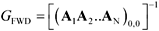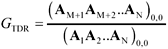# Complicated Configurations

Two-port matrices may be cascaded ad infinitum to create structures of arbitrary complexity. Presuming a number of two-port sections A 1 , A 2 ... A N have been cascaded, you may calculate the overall circuit gain.

Equation C.20The various sections could represent, for example,

• A series-connected two-port representing the output impedance of the driver on an integrated circuit die: z 1 ( w ) = j w L DIE + R DIE
• A series-connected two-port representing the series impedance of a wire-bond connection to the chip package: z 2 ( w ) = j w L WIREBOND + R WIREBOND
• A shunt-connected two-port representing the wire-bond landing pad capacitance ,• A transmission-line two-port representing the characteristic impedance, delay, and loss of the BGA routing track
• A series-connected two-port representing the BGA ball inductance
• A shunt-connected two-port representing the BGA ball capacitance
• A series of three two-port models: shunt, series, and shunt, representing a pi-model of the pcb via
• A transmission-line two-port representing a skinny breakout track as it winds its way out of the BGA ball field
• A transmission-line two-port representing a regular track proceeding a long distance towards a receiver
• A series of three two-port models: shunt, series, and shunt, representing a pi-model of an intermediate pcb via,

...and so on until the model is sufficiently rich to satisfy your desire for accuracy.

The TDR response of a complicated two-port model involving N stages, defined as the gain from the input voltage source on the left to a point just to the right of (after) stage M, is

Equation C.21High-Speed Signal Propagation[c] Advanced Black Magic
ISBN: 013084408X
EAN: N/A
Year: 2005
Pages: 163